#### Cm to inches and inches to cm converter

In this Excel tutorial lesson, you will learn how to convert cm to inches, cm to feet and inches and inches to cm using the Microsoft Excel application.

This is a common question to convert between metric and imperial units. Excel can do that in various different ways. Let’s convert cm to inches and inches to cm using Excel.

What is 1 inch equal to in cm?

1 inch is equal to 2.54 cm.

5,9 inches is equal to 5,9 * 2.54cm = 14.986cm

What is 1 cm equal to in inches?

1 centimeter is equal to 0.3937007874015748 inches.

47 cm = 47 / 2.54inches =  18.50393700787402 inches

## Method 1. Convert using simple division

2.54 is the crucial number here. To convert cm to inches, you need to just divide by 2.54. Similarly, to convert inches to cm, you need to multiply by 2.54.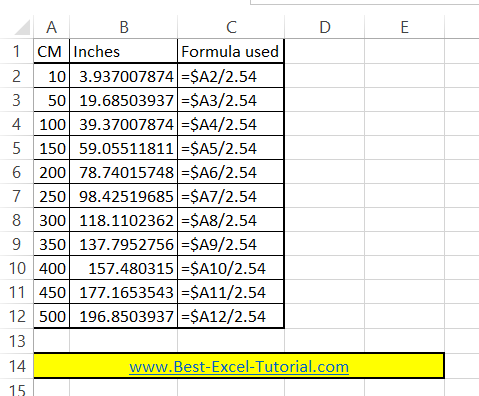Examples:

10 inches = 10 * 2.54cm = 25.4cm.

10 cm = 10 / 2.54inches = 3.937007874015748 inches.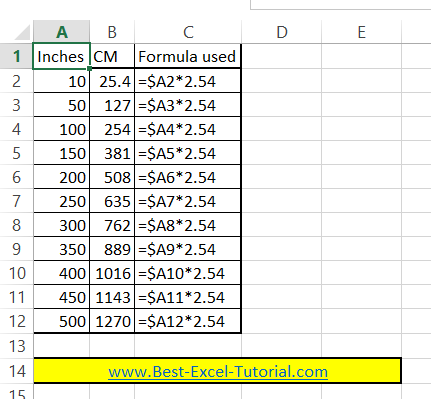Note: You may want to round the inch value for simplification. Just use the ROUND Excel function.

10 cm = ROUND(10/2.54,1) = 3.9 inches

10 cm = ROUND(10/2.54,2) = 3.94 inches

10 cm = ROUND(10/2.54,5) = 3.93701 inches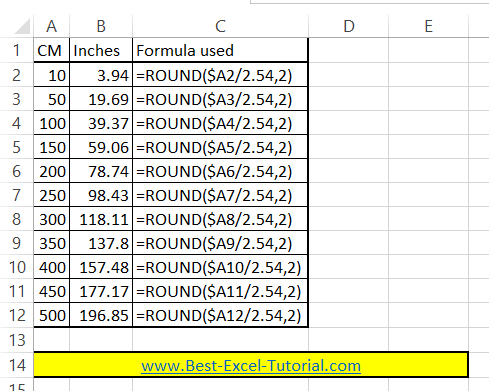## Method 2. Conversion using CONVERT Excel function

The CONVERT function automatically converts the value based on the specified input and output units, which eliminates the need for a separate conversion factor or formula. This method is more efficient and less prone to errors, as the conversion is handled by the built-in function in Excel.

=CONVERT(cell,”unit1″,”unit2″)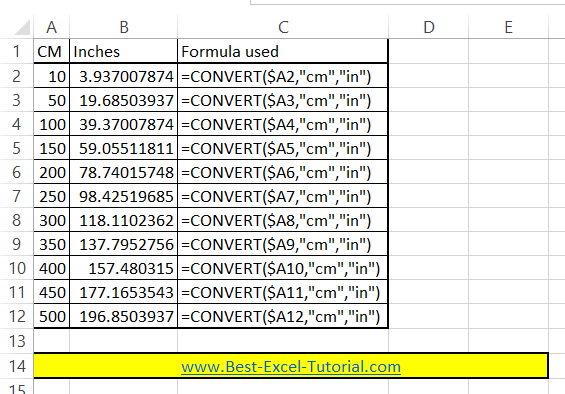To convert cm to inches use:

=CONVERT(cell,”cm”,”in”)

To convert inches to cm use:

=CONVERT(cell,”in”,”cm”)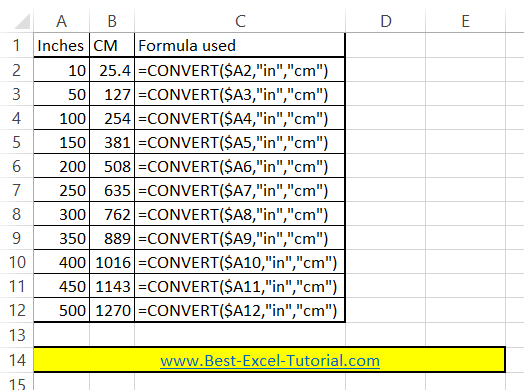## Method 3. Convert using VBA code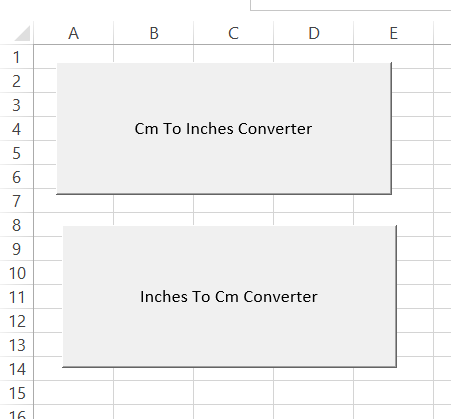This VBA code will ask you how many inches you want to convert to cm:```Sub InchesToCmConverter()
Dim InchesToCm As Long
InchesToCm = InputBox("How many in Inches?")
MsgBox InchesToCm * 2.54 & " cm."
End Sub```

MsgBox will show you the answer.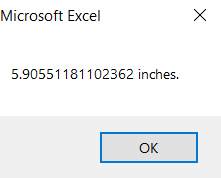This VBA code will ask you how many cm you want to convert to inches:

``````Sub CmToInchesConverter()
Dim CmToInches As Long
CmToInches = InputBox("How many in Cm?")
MsgBox CmToInches / 2.54 & " inches."
End Sub``````

MsgBox will show you the answer.

The custom functions in the Visual Basic module handle the conversion by applying the appropriate formula, which makes the conversion process easier and more efficient. This method also allows you to modify the conversion formulas if needed, by simply editing the code in the module.

## Method 4. Convert cm to feet and inches.

1 meter is equal to 100 cm to it is obvious to calculate. One foot is 12 inches, making it more difficult to calculate. Luckily, there is an Excel formula which converts cm to feet and inches.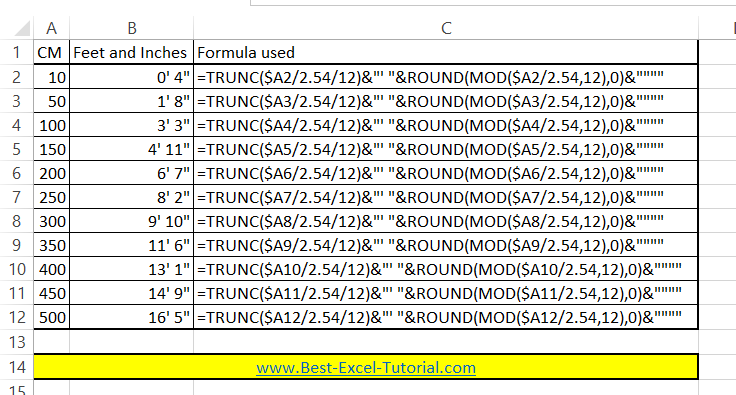=TRUNC(A1/2.54/12)&”‘ “&ROUND(MOD(A1/2.54,12),0)&””””

It will convert 180 cm to 5′ 11″

Feel free to download a my GitHub account with the above methods.